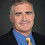## Thursday, April 16, 2015

### PH Infinite Under a Random Oracle

Benjamin Rossman, Rocco Servedio and Li-Yang Tan show new circuit lower bounds that imply, among other things, that the polynomial-time hierarchy is infinite relative to a random oracle. What does that mean, and why is it important?

The polynomial-time hierarchy can be defined inductively as follows: ΣP= P, the set of problems solvable in polynomial-time. ΣPi+1 = NPΣPi, the set of problems computable in nondeterministic polynomial-time that can ask arbitrary questions to the previous level. We say the polynomial-time hierarchy is infinite if ΣPi+1 ≠ ΣPi for all i and it collapses otherwise.

Whether the polynomial-time hierarchy is infinite is one of the major assumptions in computational complexity and would imply a large number of statements we believe to be true including that NP-complete problems do not have small circuits and that Graph Isomorphism is not co-NP-complete.

We don't have the techniques to settle whether or not the polynomial-time hierarchy is infinite so we can look at relativized worlds, where all machines have access to the same oracle. The Baker-Gill-Solovay oracle that makes P = NP also collapses the hierarchy. Finding an oracle that makes the hierarchy infinite was a larger challenge and required new results in circuit complexity.

In 1985, Yao in his paper Separating the polynomial-time hierarchy by oracles showed that there were functions that had small depth d+1 circuits but large depth d circuits which was needed for the oracle. Håstad gave a simplified proof. Cai proved that PSPACE ≠ ΣPi for all i even if we choose the oracle at random (with probability one). Babai later and independently gave a simpler proof.

Whether a randomly chosen oracle would make the hierarchy infinite required showing the depth separation of circuits in the average case which remained open for three decades. Rossman, Servedio and Tan solved that circuit problem and get the random oracle result as a consequence. They build on Håstad's proof technique of randomly restricting variables to true and false. Rossman et al. generalize to a random projection method that projects onto a new set of variables. Read their paper to see all the details.

In 1994, Ron Book showed that if the polynomial-time hierarchy was infinite that it remained infinite relativized to a random oracle. Rossman et al. thus gives even more evidence to believe that the hierarchy is indeed infinite, in the sense that if they had proven the opposite result than the hierarchy would have collapsed.

I used Book's paper to show that a number of complexity hypothesis held simultaneously with the hierarchy being infinite, now a trivial consequence of the Rossman et al. result. I can live with that.

1."showed that there were functions that had small depth d+1 circuits but large depth d circuits which was needed for the oracle."

I really don't understand this sentence. What was "needed for the oracle"? How does this result about bounded depth circuits relates to oracles?

1.You should think of an input to the circuit corresponding to whether a string is in the oracle. Chapter 7 of Håstad's PhD Thesis works out this connection in full detail.

2.What is known about constructive randomness, such as Martin-Löf random oracles?

1.All the random oracle results about complexity classes, including this one, hold for all Martin-Löf random oracles.

3.I can see why this could let us know that if the poly hierarchy does collapse, then we're going to have to be a bit more clever in showing how it does...but I still don't have much intuition on why this gives us evidence that the poly hierarchy is infinite.

That is, since the random oracle hypothesis is false, why does this tell us anything about the poly hierarchy being infinite?
The fact that IP is separated from PSPACE via a random oracle with probability 1, makes me lose all trust in in oracles saying something is separate.
Is there an intuition I'm missing? Or is this just a nice result in piecing together the landscape of the poly hierarchy?